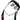Yet another blog of Matthew Lee 👀
Full-Stack Developer, good at Android 🤖️

# 深入理解达夫设备

March 21, 2020

// 正常情况下的 for 循环，
// 需要连续迭代 100000000 次
int sum = 0;
for (int i = 0; i < 100000000; i++) {
sum += i;
}

// 每次循环展开 5 次，
// 只用迭代 20000000 次即可
int sum = 0;
for (int i = 0; i < 100000000; i += 5) {
sum += i;
sum += i + 1;
sum += i + 2;
sum += i + 3;
sum += i + 4;
}

send(to, from, count)
register short *to, *from;
register count;
{
do
*to = *from++;
while (--count > 0);
}

（显然，当 count 为 0 时就会停止运行）

VAX 的 C 编译器会将上述循环编译为两条指令（我认为分别是 movw 指令和 sobleq 指令）。事实证明，这段程序正是实时播放动画程序的瓶颈，大概慢了 50%。标准的处理方式是通过循环展开，减少 sobleq 指令的调用次数，从而取得更高的速度。当你这样做时，你还得处理循环结束后剩下的那部分数据（译者注，循环次数不一定能被展开次数整除，所以需要额外处理整除不尽的余数）。我的习惯是把循环展开的语句拷贝一份作为 switch 语句。当然啦，如果我是写汇编的话，我会选择直接跳转到循环展开的语句中。一想到这里，我便在昨天写出了如下实现：

send(to, from, count)
register short *to, *from;
register count;
{
register n = (count + 7) / 8;
switch (count % 8) {
case 0: do { *to = *from++;
case 7:      *to = *from++;
case 6:      *to = *from++;
case 5:      *to = *from++;
case 4:      *to = *from++;
case 3:      *to = *from++;
case 2:      *to = *from++;
case 1:      *to = *from++;
} while (--n > 0);
}
}

send(to, from, count)
register short *to, *from;
register count;
{
register n = (count + 7) / 8;

// 循环次数不一定能被展开次数整除，
// 所以需要额外处理整除不尽的余数；
// 此处 switch 没有 break 语句，
// 会自动从余数匹配的 case 向下遍历执行（falls through）
switch (count % 8) {
case 0: *to = *from++;
case 7: *to = *from++;
case 6: *to = *from++;
case 5: *to = *from++;
case 4: *to = *from++;
case 3: *to = *from++;
case 2: *to = *from++;
case 1: *to = *from++;
}

// 每次循环展开 8 次
while (--n > 0) {
*to = *from++;
*to = *from++;
*to = *from++;
*to = *from++;
*to = *from++;
*to = *from++;
*to = *from++;
*to = *from++;
}
}